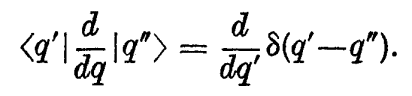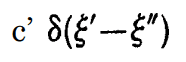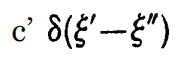# Solving the Representation of d/dq: Mistake in Reasoning?

• I
• Spin One
In summary, Dirac deduced that the representation of d/dq in "matrix" form for a system with one degree of freedom is a scalar matrix in the q representation. However, this contradicts the quantum condition pq-qp=iħ. The mistake in this line of reasoning was assuming that the derivative of the delta function is of the same form as the delta function itself. In reality, the derivative has different properties and its representation as a "matrix" would have only two diagonals with non-zero values.f

#### Spin One

When considering the position representation of a system with one degree of freedom endowed with canonical co-ordinates and momenta q and p, Dirac deduces that:which is the representation of d/dq in "matrix" form. But the derivative of a delta function is, I assume (from the definition of the delta function), of the form, with ξ' and ξ" interchanged for q' and q", since the gradient of the delta function will be 0 everywhere except at q'=q", where it will be undefined.
So the representation of d/dq is a scalar "matrix" in the q representation(from the definition)
But the momentum operator is just iħd/dq, meaning it is a scalar matrix too.
q is of course also a scalar matrix (since the representation is built upon it), and since scalar matrices commute, we get that:
pq-qp=0, which contradicts the quantum condition pq-qp=iħ.

There must be a mistake in my line of reasoning, and I would much appreciate it if someone can point it out.

#### Attachments

But the derivative of a delta function is, I assume (from the definition of the delta function), of the form, with ξ' and ξ" interchanged for q' and q", since the gradient of the delta function will be 0 everywhere except at q'=q", where it will be undefined.
This is an incorrect assumption. The derivative of the delta distribution is different from the delta distribution itself. Instead of having the property
$$\int f(x) \delta(x) dx = f(0)$$
as the delta distribution, it has the property
$$\int f(x) \delta'(x) dx = - \int f'(x) \delta(x) dx = -f'(0).$$

#### Attachments

•bhobba
This is an incorrect assumption. The derivative of the delta distribution is different from the delta distribution itself. Instead of having the property
$$\int f(x) \delta(x) dx = f(0)$$
as the delta distribution, it has the property
$$\int f(x) \delta'(x) dx = - \int f'(x) \delta(x) dx = -f'(0).$$
Oh ok, thanks! I see now how my intuition was wrong, since taking the δ function in the sense of a limit of a proper function, the derivative must be 0 at δ(0), +∞ at δ(-ε) and -∞ at δ(+ε). In that case, will the representation of d/dq be a "matrix" (of the generalized kind) with only two diagonals just above and just below the main diagonal having a non-zero value?

Last edited: